# Uniform White Noise VI

## LabVIEW 2018 Help

Edition Date: March 2018
Part Number: 371361R-01
View Product InfoLabVIEW 2016 HelpLabVIEW 2017 HelpLabVIEW 2018 HelpLabVIEW 2019 HelpLabVIEW 2020 Help

Owning Palette: Signal Generation VIs

Requires: Full Development System

Generates a uniformly distributed, pseudorandom pattern whose values are in the range [–a:a], where a is the absolute value of amplitude.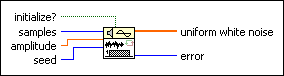initialize? controls the reseeding of the noise sample generator. LabVIEW maintains the internal seed state of this VI. If initialize? is TRUE, this VI updates the internal seed state according to the value of seed. If initialize? is FALSE, this VI resumes producing noise samples as a continuation of the previous noise sequence. The default is TRUE.samples is the number of the samples of the uniform white noise. samples must be greater than 0. The default is 128.amplitude is the amplitude of uniform white noise. The default is 1.0.seed determines how to generate the internal seed state when initialize? is TRUE. If seed is greater than 0, this VI uses seed to generate the internal state directly. If seed is less than or equal to 0, this VI uses a random number to generate the internal state. If initialize? is FALSE, this VI ignores seed. The default is -1.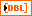uniform white noise contains the uniformly distributed, pseudorandom pattern.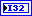error returns any error or warning from the VI. You can wire error to the Error Cluster From Error Code VI to convert the error code or warning into an error cluster.

## Uniform White Noise Details

The Uniform White Noise VI generates the pseudorandom sequence using a modified version of the Very-Long-Cycle random number generator algorithm. The pseudorandom number generator implements a triple-seeded linear congruential algorithm. Given that the probability density function, f(x), of the uniformly distributed uniform white noise is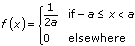,

where a is the absolute value of the specified amplitude.

The following equations define the expected mean value, µ, and the expected standard deviation value,, of the pseudorandom sequence:

µ = E{x} = 0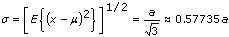The pseudorandom sequence produces approximately 290 samples before the pattern repeats itself.

You can use uniform white noise as a stimulus to measure the frequency response of amplifiers and electronic filters.

You can use the initialize? input to generate a long random noise sequence block by block. The following block diagram shows two ways to generate identical 300-sample uniform white noise sequences with a seed of 2.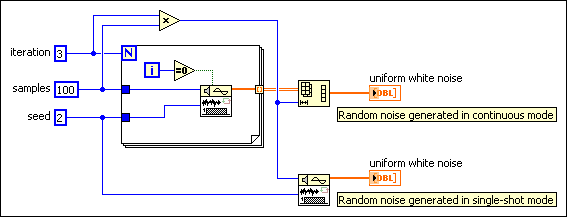You also can use the Uniform White Noise Waveform VI to generate a uniform white noise signal or the Continuous Random VI to generate random values from a continuous uniform-distributed variate.